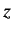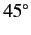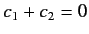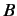# Ket Space

Consider a microscopic system composed of particles or bodies with specific properties (mass, moment of inertia, etc.) interacting according to specific laws of force. There will be various possible motions of the particles or bodies consistent with the laws of force. Let us term each such motion a state of the system. According to the principle of superposition of states, any given state can be regarded as a superposition of two or more other states. Thus, states must be related to mathematical quantities of a kind which can be added together to give other quantities of the same kind. The most obvious examples of such quantities are vectors.

Let us consider a particular microscopic system in a particular state, which we label $$A$$: e.g., a photon with a particular energy, momentum, and polarization. We can represent this state as a particular vector, which we also label $$A$$, residing in some vector space, where the other elements of the space represent all of the other possible states of the system. Such a space is called a ket space (after Dirac). The state vector $$A$$ is conventionally written

$$\mid A\rangle \tag{1}$$

Suppose that state $$A$$ is, in fact, the superposition of two different states, $$B$$ and $$C$$. This interrelation is represented in ket space by writing

$$\mid A\rangle = \mid B\rangle + \mid C\rangle \tag{2}$$

where $$\mid B \rangle$$ is the vector relating to the state $$B$$, etc. For instance, state $$\mid B \rangle$$ might represent a photon propagating in the-direction, and plane polarized in the $$x$$,-direction, and state $$\mid B \rangle$$ might represent a similar photon plane polarized in the $$y$$-direction. In this case, the sum of these two states represents a photon whose plane of polarization makes an angle ofwith both the $$x$$- and $$"y$$-directions (by analogy with classical physics). This latter state is represented by $$\mid B \rangle + \mid C \rangle$$ in ket space.

Suppose that we want to construct a state whose plane of polarization makes an arbitrary angle $$\alpha$$, with the $$x$$,-direction. We can do this via a suitably weighted superposition of states $$B$$, and $$C$$. By analogy with classical physics, we require $$\cos(\alpha)$$ of state $$B$$, and $$\sin(\alpha)$$ of state $$C$$. This new state is represented by

$$\cos(\alpha)\mid B\rangle + sin(\alpha)\mid C\rangle \tag{3}$$

in ket space. Note that we cannot form a new state by superposing a state with itself. For instance, a photon polarized in the $$y$$,-direction superposed with another photon polarized in the $$y$$,-direction (with the same energy and momentum) gives the same photon. This implies that the ket vector

$$c_1\mid A\rangle + c_2\mid A\rangle = (c_1 + c_2)\mid A\rangle \tag{4}$$

corresponds to the same state that $$\mid A \rangle$$ does. Thus, ket vectors differ from conventional vectors in that their magnitudes, or lengths, are physically irrelevant. All the states of the system are in one to one correspondence with all the possible directions of vectors in the ket space, no distinction being made between the directions of the ket vectors $$\mid A \rangle$$ and $$-\mid A \rangle$$. There is, however, one caveat to the above statements. Ifthen the superposition process yields nothing at all: i.e., no state. The absence of a state is represented by the null vector $$\mid 0 \rangle$$ in ket space. The null vector has the fairly obvious property that

$\mid A\rangle + \mid 0\rangle = \mid A\rangle \tag{5}$

for any vector $$\mid A \rangle$$. The fact that ket vectors pointing in the same direction represent the same state relates ultimately to the quantization of matter: i.e., the fact that it comes in irreducible packets called photons, electrons, atoms, etc. If we observe a microscopic system then we either see a state (i.e., a photon, or an atom, or a molecule, etc.) or we see nothing--we can never see a fraction or a multiple of a state. In classical physics, if we observe a wave then the amplitude of the wave can take any value between zero and infinity. Thus, if we were to represent a classical wave by a vector, then the magnitude, or length, of the vector would correspond to the amplitude of the wave, and the direction would correspond to the frequency and wave-length, so that two vectors of different lengths pointing in the same direction would represent different wave states.

We have seen, in Eq. (3), that any plane polarized state of a photon can be represented as a linear superposition of two orthogonal polarization states in which the weights are real numbers. Suppose that we want to construct a circularly polarized photon state. Well, we know from classical physics that a circularly polarized wave is a superposition of two waves of equal amplitude, plane polarized in orthogonal directions, which are in phase quadrature. This suggests that a circularly polarized photon is the superposition of a photon polarized in the $$x$$-direction (state $$B$$) and a photon polarized in the $$y$$-direction (state $$C$$, with equal weights given to the two states, but with the proviso that state $$C$$ isout of phase with state. By analogy with classical physics, we can use complex numbers to simultaneously represent the weighting and relative phase in a linear superposition. Thus, a circularly polarized photon is represented by

$\mid B\rangle + i\mid C\rangle \tag{6}$

in ket space. A general elliptically polarized photon is represented by

$c_1\mid B\rangle + c_2\mid C\rangle \tag{7}$

where $$c_1$$ and $$c_2$$ are complex numbers. We conclude that a ket space must be a complex vector space if it is to properly represent the mutual interrelations between the possible states of a microscopic system.

Suppose that the ket $$\mid R \rangle$$ is expressible linearly in terms of the kets $$\mid A \rangle$$ and $$\mid B \rangle$$, so that

$\mid R\rangle=c_1\mid A\rangle + c_2\mid B\rangle \tag{8}$

We say that $$\mid R \rangle$$ is dependent on $$\mid A \rangle$$ and $$\mid B \rangle$$. It follows that the state $$R$$ can be regarded as a linear superposition of the states $$A$$ and $$B$$. So, we can also say that state $$R$$ is dependent on states $$\mid A \rangle$$ and $$\mid B \rangle$$. In fact, any ket vector (or state) which is expressible linearly in terms of certain others is said to be dependent on them. Likewise, a set of ket vectors (or states) are termed independent if none of them are expressible linearly in terms of the others.

The dimensionality of a conventional vector space is defined as the number of independent vectors contained in the space. Likewise, the dimensionality of a ket space is equivalent to the number of independent ket vectors it contains. Thus, the ket space which represents the possible polarization states of a photon propagating in the-direction is two-dimensional (the two independent vectors correspond to photons plane polarized in the $$x$$- and $$y$$-directions, respectively). Some microscopic systems have a finite number of independent states (e.g., the spin states of an electron in a magnetic field). If there are $$N$$ independent states, then the possible states of the system are represented as an $$N$$-dimensional ket space. Some microscopic systems have a denumerably infinite number of independent states (e.g., a particle in an infinitely deep, one-dimensional potential well). The possible states of such a system are represented as a ket space whose dimensions are denumerably infinite. Such a space can be treated in more or less the same manner as a finite-dimensional space. Unfortunately, some microscopic systems have a nondenumerably infinite number of independent states (e.g., a free particle). The possible states of such a system are represented as a ket space whose dimensions are nondenumerably infinite. This type of space requires a slightly different treatment to spaces of finite, or denumerably infinite, dimensions.

In conclusion, the states of a general microscopic system can be represented as a complex vector space of (possibly) infinite dimensions. Such a space is termed a Hilbert space by mathematicians.